American Journal of Civil Engineering
Volume 4, Issue 6, November 2016, Pages: 306-313

Effect of Some Factors on the Dynamic Response of Reinforced Cylindrical Shell with a Hole on Elastic Supports Subjected to Blast Loading

Nguyen Thai Chung, Le Xuan Thuy

Department of Solid Mechanics, Le Quy Don Technical University, Ha Noi, Viet Nam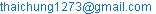(N. T. Chung)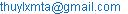(L. X. Thuy)

Nguyen Thai Chung, Le Xuan Thuy. Effect of Some Factors on the Dynamic Response of Reinforced Cylindrical Shell with a Hole on Elastic Supports Subjected to Blast Loading. American Journal of Civil Engineering. Vol. 4, No. 6, 2016, pp. 306-313. doi: 10.11648/j.ajce.20160406.16

Received: September 4, 2016; Accepted: September 13, 2016; Published: October 8, 2016

Abstract: This paper presents the finite element algorithm and calculation method of reinforced cylindrical shell with a hole under blast loading. Using the programmed algorithm and computer program written in Matlab environment, the authors solved a specific problem, from which examining the effects of structural and loading parameters to the dynamic response of the shell.

1. Introduction

Dao Huy Bich and Vu Do Long  used the analytical method to analyze the dynamics response of imperfect functionally graded material shallow shells subjected to dynamic loads. Nivin Philip, C. Prabha  analyzed static buckling of the stiffened composite cylindrical shell subjected to external pressure by the finite element method. Nguyen Thai Chung and Le Xuan Thuy  used the finite element method to analyze the dynamic of eccentrically rib-stiffened shallow cylindrical shells on flexible couplings under blast loadings. Lin Jing, Zhihua Wang, Longmao Zhao , Gabriele Imbalzano, Phuong Tran, Tuan D. Ngo, Peter V. S. Lee , Phuong Tran, Tuan D. Ngo, Abdallah Ghazlan  analyzed dynamic response of the composite shells and cylindrical sandwich shells under blast loading. Yonghui Wang, Ximei Zhai, Siew Chin Lee, Wei Wang  succeeded in analyzing the dynamic responses of curved steel-concrete-steel sandwich shells subjected to blast loading by the numerical method. Anqi Chen, Luke A. Louca and Ahmed Y. Elghazouli  analyzed dynamic behaviour of cylindrical steel drums under blast loading conditions. However, studies on the calculation of shell structure under the effect of the shock waves are few, especially of the shells with a hole.

In order to develop the study approach to the shallow cylindrical shells, in this paper, the authors set the algorithm and computer program to analyze the dynamics of rib-stiffened shallow cylindrical shells with abatement holes under the effect of the shock wave loads. Couplings on the shell borders are elastic supports with the tension- compression stiffness k.

2. Computational Model and Assumptions

Considering the eccentrically rib-stiffened shallow cylindrical shell on elastic supports, being described by springs with stiffness k. The shell is subjected to a layer shock wave. Because the shell is shallow, the shock-wave presssure affecting can be considered to be uniformly distributed over the surface of the shell (Figure 1).

The assumptions: Materials of the shell are homogeneous and isotropic; the rib and shell are linearly elastically deformed and have absolutely adhesive connection; loading process works, no cracks appearing around the hole.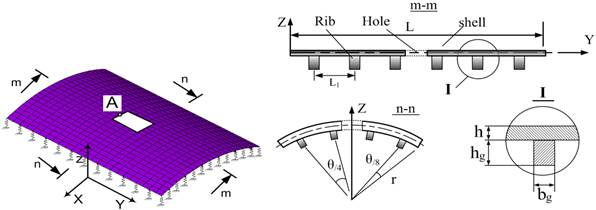Fig. 1. Problem model.

3. Finite Element Model and Basic Equations

3.1. Types of Elements to Be Used

The shell is fragmented by 4-node flat shell elements, which means that the shell is a finite combination of 4-node flat elements, is a combination of membrane elements and plate elements subject to bending and twisting combination (Figure 2).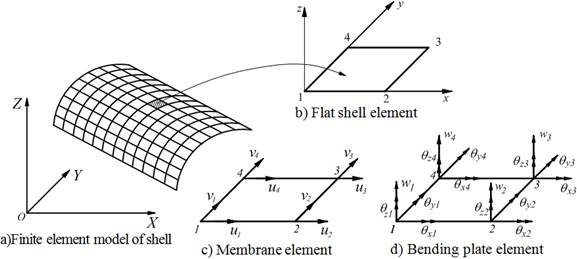Fig. 2. General shell element model.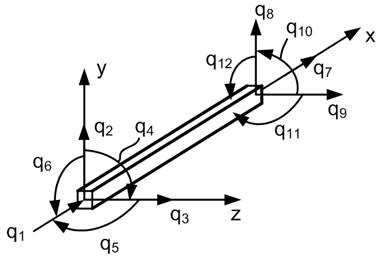Fig. 3. Beam elements.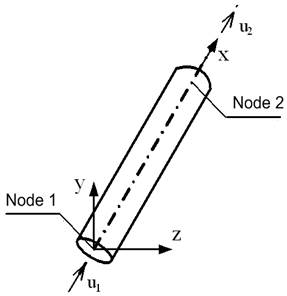Fig. 4. Bar elements.

The stiffened ribs are divided into 2-node spatial beam elements, each node has 6 degrees of freedom (Figure 3). The linearly elastic supports are described by bar elements, that are under tension and compression along its axis denoted by x, each node of the element has one degree of freedom (Figure 4) , .

3.2. Flat Shell Element Describes the Shell

Each node of the shell element is composed of 6 degrees of freedom: ui, vi, wi, qxi, qyi, qzi. Displacement of any point of the element can be written as :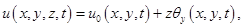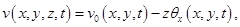(1)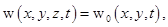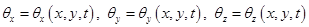where u, v, and w are the displacements along x, y and z axes, respectively; superscript "0" denotes midplane displacement; and qx, qy, and qz are rotations about the x - axis, y - axis and z - axis, respectively.

Strain vector components are: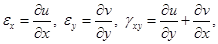(2)

Relationship stress - strain can be written as:

{σ} = [D]{ε},                                  (3)

where [D] is a matrix of relationship stress - strain.

Using Hamilton’s principle for the elements :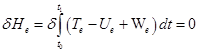(4)

where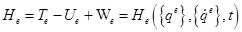is the Hamilton function, Te is the kinetic energy of the element, Ue is the total potential energy of the element, We is total external work due to mechanical loading of element e,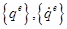are vector of nodal displacements, and vector of nodal velocities, respectively.

Considering the case not mention the damping, from (4) leads to the following:(5)

The kinetic energy Te of the elements is determined by the expression :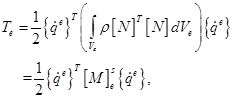(6)

where [N] is function matrix of flat shell elements , , Ve is element volume,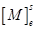is element mass matrix, ρ is specific volume of materials.

The total potential energy Ue is determined by:(7)

In which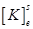is stiffness matrix of flat shell elements.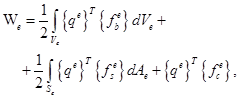(8)

with Ae is element area,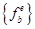- volume force vector,- surface force vector,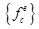- concentrated force vector of the elements , .

Substitute (6), (7), (8) into (4), (5), we have the differential equation describing the vibration of the shell element in matrix form as follow: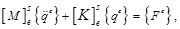(9)

where {qe} is the vector of nodal displacements, {Fe} is the mechanical force vector.

In the (X, Y, Z) coordinate system: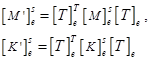(10)

[T]e is the coordinate axes transition matrix .

3.3. Space Beam Element Describes the Rib

Displacement in any node of the bar with (x, y) coordinates is identified as follows :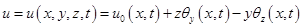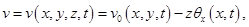(11)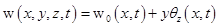where, the subscript "0" represents axis x (y = 0, z = 0), t represents time; u, v and w are the displacements along x, y and z; θx is the rotation of cross section about the longitudinal axis x; and θy and θz denote rotations of the cross section about y and z axes.

The strain components:(12)

Nodal displacement vector:

{q}eb = {q1, q2, q3, q4, q5, q6, q7, q8, q9, q10, q11, q12}T   (13)

Element stiffness matrix is set up from 4 types of component stiffness matrices , :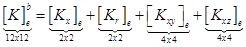(14)

where,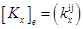,, i, j = 1, 2;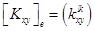,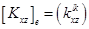, l, k = 1÷4, are tension (compression) stiffness matrix, torsion stiffness matrix, bending stiffness matrix in the xy plane, and bending stiffness matrix in the xz plane, respectively.(15)

Similarly, element mass matrix is also established from 4 types of volume matrix:(16)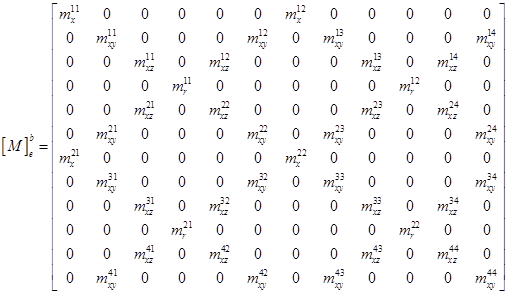(17)

In the (X, Y, Z) coordinate system:3.4. Bar Element Describes the Elastic support

Node displacement vector and stiffness matrix of bar element is :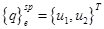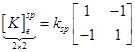(18)

where, ksp is the tension- compression stiffness of elastic support.

3.5. Governing Equations and Solving Method

The connection of bar elements and space beam elements into the flat shell elements forming the rib-stiffened shell – elastic support system is implemented by direct stiffness method and Skyline diagram under the general algorithm of Finite element method , . After connecting and getting rid of margins, the governing equations of the rib-stiffened shell – elastic support system is: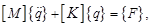(19)

In the case of taking the damping into account the equation (19) becomes: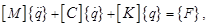(20)

where:- overall mass matrix (after getting rid of margins);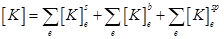- overall stiffness matrix (after getting rid of margins).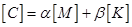- overall damping matrix, α, β are Rayleigh damping coefficients .

Equation (20) is a linear dynamic equation and may be solved by using the Newmark’s direct integration method. Based on the established algorithm the authors have written the program called Stiffened_SC_Shell_Withhole in Matlab environment.

4. Numerical Examination

4.1. The Effects of Abatement Hole

Considering the shallow cylindrical shell whose plan view is a rectangular, generating line’s length l = 3.0m, opening angle of the shell θ = 40°, the radius of curvature is r = 2.0m, shell thickness th = 0,02m. The shell material has elastic modulus E = 2.2´1011 N/m2, Poisson coefficient ν = 0.31, specific volume ρ = 7800kg/m3. The eccentrically ribbed shell with the height of ribs hg = 0.03m, thickness of ribs thg = 0.006m, the shell with 4 ribs is parallel to the generating line, 6 ribs is perpendicular to the generating line, the ribs are equispaced. The ribs’ material has E = 2.4´1011 N/m2, ν = 0.3, ρ = 7000kg/m3. Considering the problem with two cases:

Case 1: (basic problem): The shell has a square (a x a) abatement hole in the middle position, with a = 0.3 m;

Case 2: The shell has no hole (a = 0).

Acting load: the shock waves act uniformly to the direction of normal on the shell surface according to the law: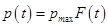,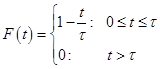, pmax = 3.104 N/m2, τ = 0.05s.

Conditions of coupling: Four sides of the shells with couplings are limited to move horizontally and leaned on elastic supports with the tension- compression stiffness k = 3.5x104 kN/m.

Case 1: The shell has a square abatement hole with the side a = 0.3 m (Basic problem):

Using the established Stiffened_SC_Shell _withhole program, the authors solved the problem with the calculating time tcal = 0.08s, integral time step Δt = 0.0005s. The results of deflection response and stress at the midpoint of the hole edge (point A) are shown in Figures 5, 6.

Case 2: The shell has no hole:

Results in Figures 7 and 8 respectively are deflection response and stress at the midpoint of the shell.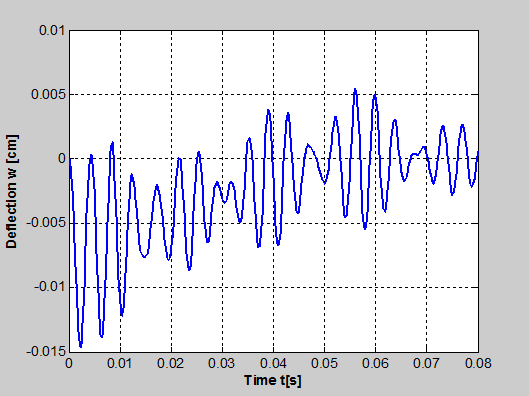Fig. 5. Displacement response w at point A.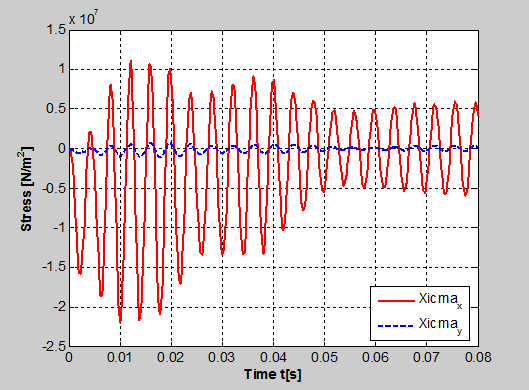Fig. 6. Stress response sx, σy at point A.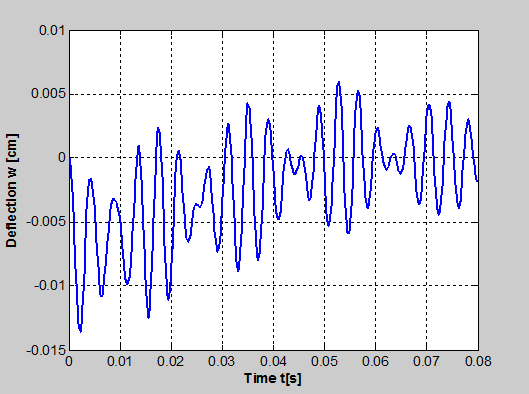Fig. 7. Displacement response w at the midpoint of the shell.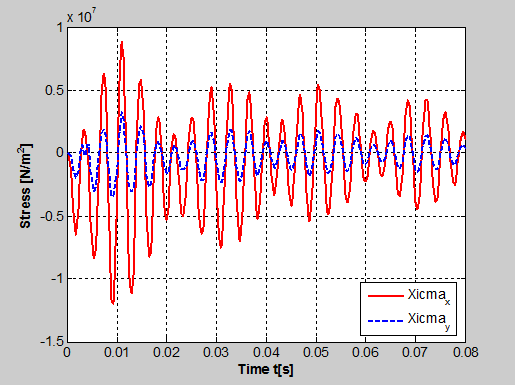Fig. 8. Stress response sx, σy at the midpoint of the shell.

Table 1. Comparison of the values of displacements and stresses in two cases.

 Deflection Wzmax [cm] Stress σxmax [N/m2] Stress σymax [N/m2] Case 1 0.01471 21.964.106 1.111.106 Case 2 0.01358 12.009.106 3.423.106

Comment: When there is a hole, both displacements and stresses in the structure are increased. Especially, the maximum stress in the structure increases rapidly. This explains the destruction vulnerability of the structure when it has defects.

4.2. The effects of the size of the hole

Examining the problem with the size of the hole changes: a1 = 0.15 m, a2 = 0.25 m, a3 = 0.30 m. Displacement response and real-time stresses at point A corresponding to cases shown in Figures 9, 10.

Table 2. Extreme values of calculated quantities at point A when the size a changes.

 a [m] Wzmax [cm] Stress σxmax [N/m2] Stress σymax [N/m2] 0.15 0.01577 20.389.106 1.212.106 0.25 0.01521 20.716.106 1.808.106 0.30 0.01471 21.964.106 1.111.106

Comment: Generally, when increasing the size of the abatement hole, point A shifts closer to the stiffening rib, so the stiffness of the area surrounding point A increases, making the displacement of point A reduces, stress increases.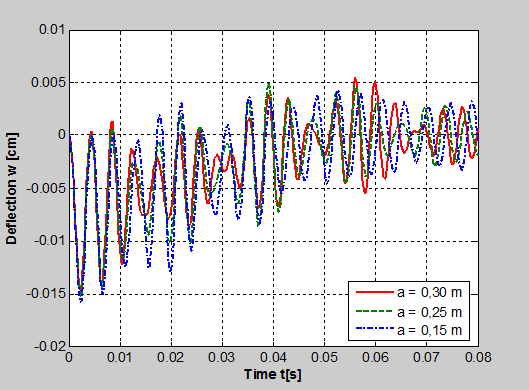Fig. 9. Deflection response w at point A based on the size a.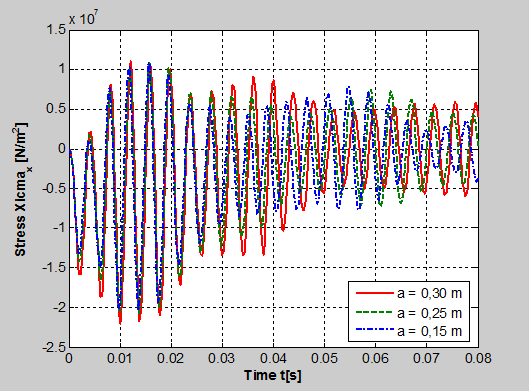Fig. 10. Stress response sx at point A based on the size a.

4.3. The Effects of Radius r

Examining the problem with r changes: r1 = 2.0 m, r2 = 2.3 m, r3 = 2.5 m, r4 = 2.8 m, r5 = 3.0 m. Extreme values of the deflection and stresses at the calculated point are expressed in table 3 and Figures 11, 12, 13, 14.Fig. 11. Deflection response w when changing r.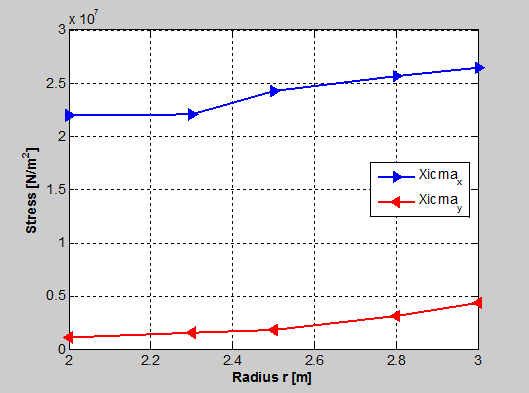Fig. 12. Stress response sx, σy when changing r.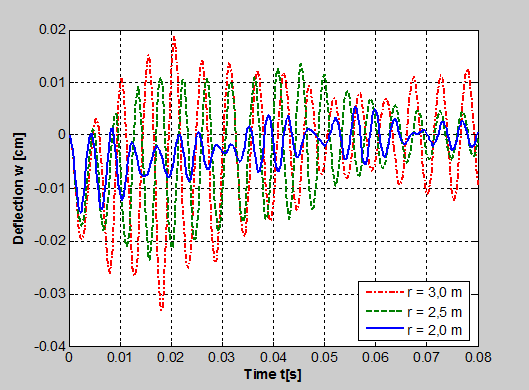Fig. 13. Deflection response w with various values of r.Fig. 14. Stress response sx with various values of r.

Table 3. Extreme values of calculated quantities at point A when the size r changes.

 r [m] Wzmax [cm] Stress σxmax [N/m2] Stress σymax [N/m2] 2.0 0.01471 21.964.106 1.111.106 2.3 0.01799 22.556.106 1.499.106 2.5 0.02361 24.284.106 1.841.106 2.8 0.02837 25.654.106 3.140.106 3.0 0.03298 26.448.106 4.340.106

Comment: When preserving the opening angle of the shell and other parameters, increasing the radius r will increase the displacement and stress at the calculated point. At this time, the vibration of the structure increases rapidly (Figure 13).

4.4. The Effects of the Height of Rib

Assessing the effects of the height of the stiffening rib, the authors examined the problem with hg changes: hg1 = 0.03 m, hg2 = 0.04 m, hg3 = 0.05 m, hg4 = 0.06 m, hg5 = 0.07 m. Displacement response and real-time stresses at point A corresponding to cases shown in Figures 15, 16, 17, 18.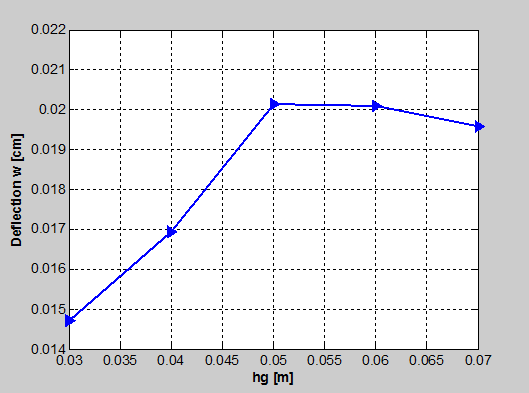Fig. 15. Deflection response w when changing hg.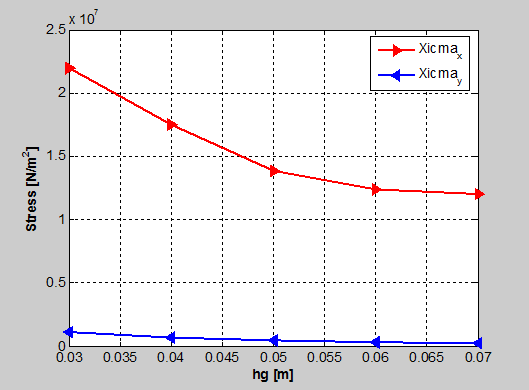Fig. 16. Stress response sx, σy when changing hg.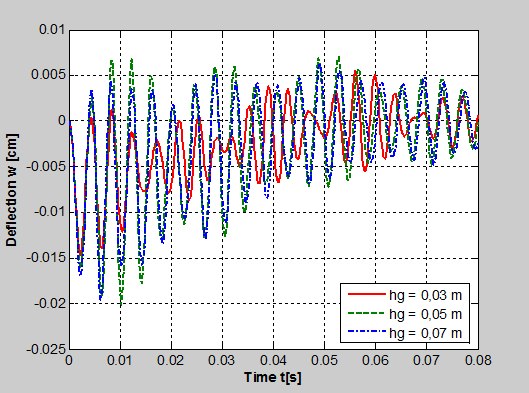Fig. 17. Deflection response w with various values of hg.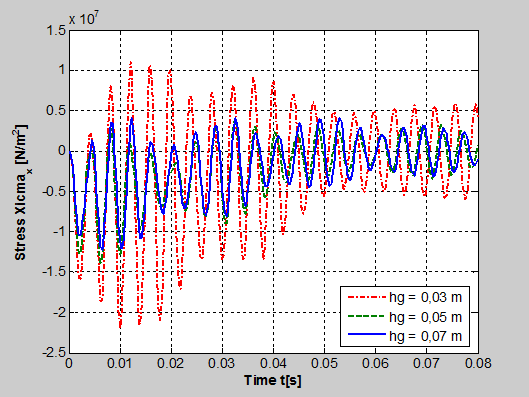Fig. 18. Stress response sx with various values of hg.

Comment: In the examined value range of hg, while increasing hg, stresses σx, σy at the calculated point reduce nonlinearly. The displacement at the initial calculated point increases (hg = 0.03m ÷ 0.05 m), then decreases (hg = 0.06m ÷ 0.07 m). This can be explained as follow: When increasing the height of rib, the stiffness of the shell increases making it less deformed. However, the shell uses the elastic seat connection, so when the stiffness of the shell increases making more load transfers to the elastic seating which leads to the increase of the total displacement of the calculated point. In phase hg = 0.06m ÷ 0.07m, after the seating shifts down fully to become a hard seating, this time, the stabler stiffness structure will make the shell less deformed, so the displacement at the calculated point reduces compared to the previous case (hg = 0.05m).

Table 4. Extreme values of calculated quantities at point A when changing the size of hg.

 hg [m] Wzmax [cm] Stress σxmax [N/m2] Stress σymax [N/m2] 0.03 0.01471 21.964.106 1.111.106 0.04 0.01694 17.487.106 0.706.106 0.05 0.02014 13.857.106 0.477.106 0.06 0.02010 12.361.106 0.340.106 0.07 0.01958 12.052.106 0.272.106

5. Conclusions

Set up the governing equations of system, finite element algorithm and computer program to analyze the dynamics of the rib-stiffened shallow shells with a holes on elastic supports under the effect of the blast loading.

Examined some structural factors such as: hole size, curve radius, height of rib, thereby making the assessment of the influence level of these factors to the dynamic response of the mentioned shell.

The results of the paper can be used as a reference for the calculation and design of similar structures, with any hole.

References

1. Dao Huy Bich, Vu Do Long (2010), Nonlinear dynamic analysis of imperfect functionally graded material shallow shells, Vietnam Journal of Mechanics, VAST, Vol.32, No.1 (2010), pp.1-14.
2. Nivin Philip, C.Prabha (2013), Numerical investigation of stiffened composite cylindrical shell subjected to external pressure, International Journal of Emerging technology and Advanced Engineering, volume 3, issue 3, March 2013, pp.591-598.
3. Nguyen Thai Chung, Le Xuan Thuy (2015), Analysis of the Dynamics of Eccentrically Rib-stiffened shallow cylindrical shells on Flexible Couplings under the effect of the blast loadings, Journal of Construction, No. 4.2015, Viet Nam, pp.73-76.
4. Lin Jing, Zhihua Wang, Longmao Zhao (2013), Dynamic response of cylindrical sandwich shells with metallic foam cores under blast loading – Numerical simulations, Composite Structures 99 (2013), pp.213-223.
5. Gabriele Imbalzano, Phuong Tran, Tuan D. Ngo, Peter V.S. Lee (2016), A numerical study of auxetic composite panels under blast loadings, Composite Structures 135(2016), pp.339-352.
6. Phuong Tran, Tuan D. Ngo, Abdallah Ghazlan (2016), Numerical modelling of hybrid elastomeric composite panels subjected to blast loadings, Composite Structures 153(2016), pp.108-122.
7. Yonghui Wang, Ximei Zhai, Siew Chin Lee, Wei Wang (2016), Responses of curved steel-concrete-steel sandwich shells subjected to blast loading, Thin-Walled Structures 108(2016), pp.185-192.
8. Anqi Chen, Luke A. Louca, Ahmed Y. Elghazouli (2016), Behaviour of cylindrical steel drums under blast loading conditions, International Journal of Impact Engineering 88(2016), pp.39-53.
9. O. C. Zienkiewicz, Taylor R. L. (1998), The Finite Element Method, McGraw-Hill, International Edition.
10. Young W.Kwon, Hyochoong Bang (1997), The finite element method using Matlab, CRC mechanical engineering series.
11. Nguyen Thai Chung, Hoang Hai, Shin Sang Hee (2016), Dynamic Analysis of High Building with Cracks in Column Subjected to Earthquake Loading, American Journal of Civil Engineering, 2016; 4(5), pp.233-240.
12. (2006), Advanced Dynamics of Structures, NTUST - CT 6006.

 Contents 1. 2. 3. 3.1. 3.2. 3.3. 3.4. 3.5. 4. 4.1. 4.2. 4.3. 4.4. 5.
Article ToolsAbstractPDF(497K)